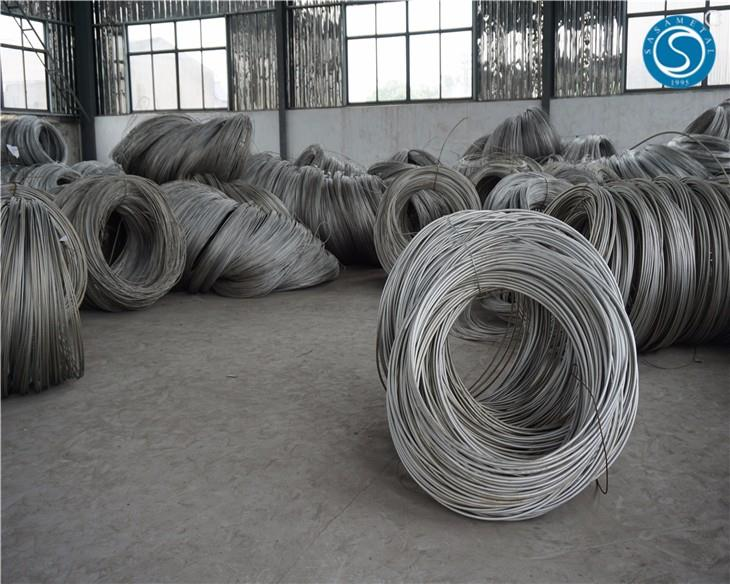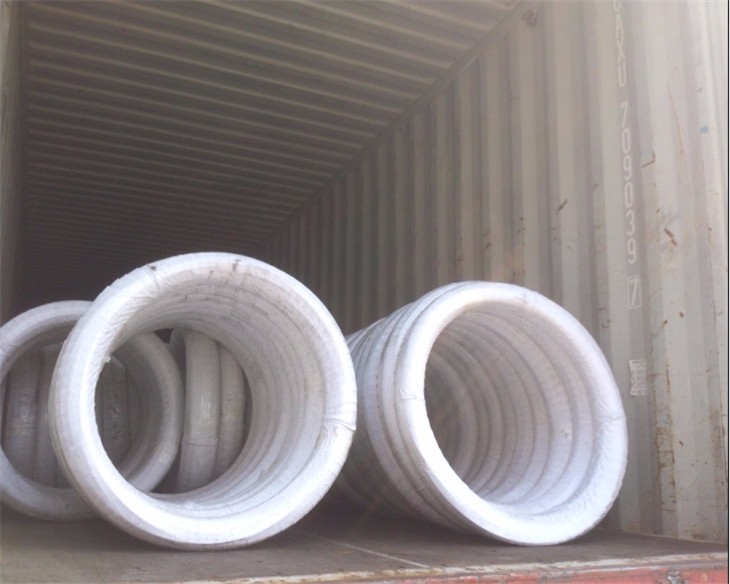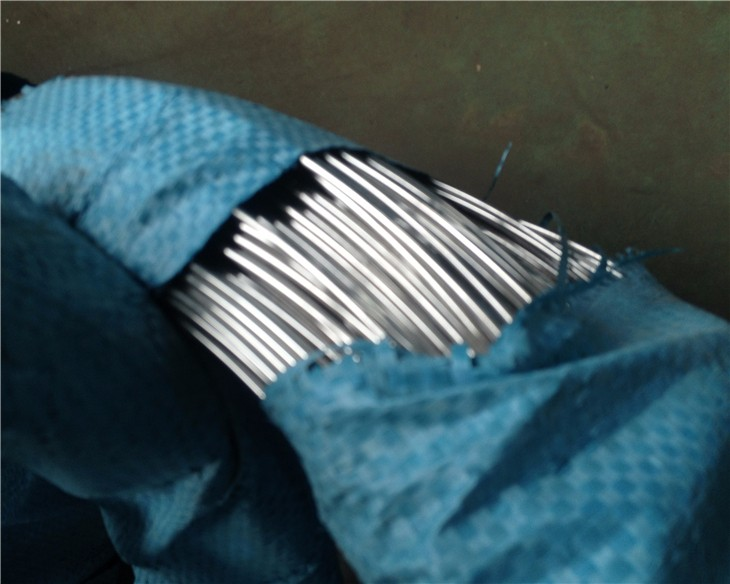# 304 Stainless Steel Wire Rod

• FOB Price: US \$0.5 - 9,999 / Piece
• Min.Order Quantity: 100 Piece/Pieces
• Supply Ability: 10000 Piece/Pieces per Month
• Port: Shanghai Ningbo Shenzhen
• Payment Terms: T/T , L/C

## Product Tags

304 stainless steel wire rod

 C% Si% Mn% P% S% Cr% Ni% N% Mo% Cu% =0.08 =1.0 =2.0 =0.045 =0.03 18.0-20.0 8.0-10.0 – - –
 T*S Y*S Hardness Elongation (Mpa) (Mpa) HRB HB (%) =520 =205 – – =40

stainless steel wire Rod products from  Sakysteel :304 stainless steel wire Rod manufacturers304 stainless steel wire Rod suppliers

More details of 304 stainless steel wire rod

A) Material Classification:
200 series: 201, 202
300 series: 01,302,304,304L,304H,309S,310S,316,316L,321,347…
400 series: 410,420,430,431,434…
B) Hardness Classification:
Soft | 1/4H | 1/2H | 3/4H | H | EH |SH
C) Surface Classification:
Bright; Gray; Oxidation; Burnishing; Coppering; Lead plating and so on
D) Purpose Classification:
For spring; cold upsetting; welding; wire rope
E )Physical Classification:
Magnetic / Nonmagnetic
F) Shape classification:
Round wire; Half round wire; Square wire; pressure flat wire; precision shaped wire

Grades of stainless steel wire rod

 Grade C Si Mn Cr N P S Ni Cu Ti Mo 201 =0.15 =0.75 5.5~7.50 16.0~18.0 =0.25 =0.06 =0.03 3.5~5.5 0.80% 202 =0.15 =1.0 7.50~10.0 17.0~19.0 =0.25 =0.06 =0.03 4.0~6.0 – 301 =0.15 =1.0 =2.0 16.0~18.0 – =0.045 =0.03 6.0~8.0 – 302 =0.15 =1.0 =2.0 17.0~19.0 – =0.035 =0.03 8.0~10.0 – 302HQ =0.15 =1.0 =2.0 8.0~10.0 – =0.045 =0.03 8.0~10.0 3~4 304 =0.08 =1.0 =2.0 18.0~20.0 – =0.045 =0.03 8.0~11.0 – 304L =0.03 =1.0 =2.0 18.0~20.0 – =0.045 =0.03 8.0~11.0 – 304H 0.04~0.1 =1.0 =2.0 18.0~20.0 – =0.045 =0.03 8.0~11.0 – 304N =0.08 =0.75 =2.0 18.0~20.0 0.1~0.16 =0.045 =0.03 8.0~11.0 – 316 =0.08 =1.0 =2.0 16~18.0 – =0.035 =0.03 10.0~14.0 – 2.0~3.0 316L =0.03 =1.0 =2.0 16.0~18.0 – =0.045 =0.03 10.0~14.0 – 2.0~3.0 321 =0.08 =1.0 =2.0 17.0~19.0 – =0.045 =0.03 9.0~12.0 – =0.7 410 =0.15 =1.0 =1 11.5~13.5 – =0.040 =0.03 – – 420 0.3~0.4 =1.0 =1 12.0~14.0 – =0.040 =0.03 =0.75 – 430 =0.12 =0.75 =1 16.0~18.0 – =0.040 =0.03 =0.60 –
 AISI,ASTM JIS EN Spring Wire Forming Wire EPQ Wire CHQ Wire Weaving Wire Anealed Wire Flat Wire 201,S20100 SUS201 1.4372 v v v v v v 202,S20200 SUS202 1.4373 v v v 301,S30100 SUS301 1.4310 v v v 302,S30200 SUS302 1.4300 v v v 302HQ SUS302HQ 1.4567 v v v v 304,S30400 SUS304 1.4301 v v v v v v 304L,S30403 SUS304L 1.4306 v v v 304H SUS304H 1.4948 v v v 304N,S30451 SUS304N1 1.4315 v v 316,S31600 SUS316 1.4401 v v v v v v 316L,S31603 SUS316L 1.4435 v v v v 321,S32100 SUS321 1.4878 v v v 410,S41000 SUS410 1.4006 v v v 420,S42000 SUS420J1 1.4021 v v 430,S43000 SUS430 1.4016 v v v v

304 stainless steel wire Rod Packaging:

Sakysteel 304 stainless steel wire Rod are packed and labeled according to the regulations and customer’s requests. Great care is taken to avoid any damage which might otherwise be caused during storage or transportation.304 stainless steel wire Rod for sale304 stainless steel wire Rod price

• Previous:
• Next: